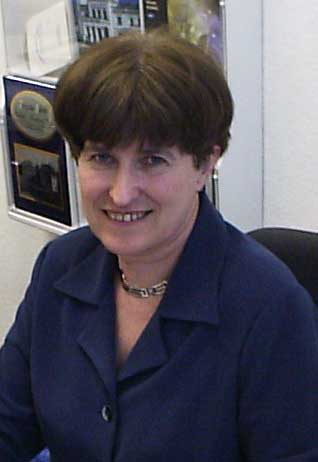Teresa Regińska, professor Institute of Mathematics, Polish Academy of Sciences email Śniadeckich 8, 00-656 Warsaw, Poland phone (office): +48 22 52 28 112

 Ph. D.: IM PAN 1975, habilitation: IM PAN 1987

### Fields of interest:

numerical analysis in abstract spaces, numerical methods for differential equations,
ill-posed problems, inverse problems for differential equations,
regularization methods, choice rules for regularization parameters.

### Selected publications:

1. Sideways heat equation and wavelets, Journal of Computational and Applied Mathematics, vol.63 (1995), 209–214.
2. A regularization parameter in discrete ill-posed problems, SIAM J. Sci. Comput. vol.17, No.3 (1996), 740–749.
3. (with L. Eldén) Solving the sideways heat equation by a wavelet-Galerkin method, Inverse Problems 13 (1997), 1093–1106.
4. (with L. Eldén and F. Berntsson) Wavelet and Fourier methods for solving the sideways heat equation, SIAM J. Sci. Comput. 21, No.6 (2000), 2187–2205.
5. (with L. Eldén) Stability and convergence of wavelet-Galerkin method for sideways heat equation, J. Inverse and Ill-Posed Problems 8, no.1 (2000), 31–49
6. Application of wavelet shrinkage to solving sideways heat equation, BIT 41, no 5 (2001), 1101–1110.
7. Regularization of discrete ill-posed problems, BIT Numerical Mathematics 44 (2004), 119–133.
8. (with A. Wakulicz) Wavelet moment method for Cauchy problem for the Helmholtz equation, Journal of Comp. and Appl. Math. 223 (2009), 218–229,
9. (with K.Regiński) Approximate solution of a Cauchy problem for the Helmholtz equation, Inverse Problems 22 (2006), 975–989.
10. (with W. Arendt) An ill-posed boundary value problem for the Helmholtz equation on Lipschitz domain, Journal of Inverse and Ill-Posed Problems 17 (2009) 703-711
11. (with U. Tautenhahn) Conditional stability estimates and regularization with applications to Cauchy problems for the Helmholtz equation, Numerical Functional Analysis and Optimization, 30 (2009), 1065-1097.
12. Two-parameter discrepancy principle for combined projection and Tikhonov regularization of ill-posed problems, J. Inverse Ill-Posed Probl. 21 (2013) 561-577
13. (with K. Regiński) Regularization strategy for determining laser beam quality parameters , J. Inverse Ill-Posed Probl. 23 (2015), 657-671.
14. Discrepancy sets for combined LSQ projection and Tikhonov regularization, MMA 22 (2017) 202-212
15. (with K. Regiński) Modification of Iterative Tikhonov Regularization Motivated by a Problem of Identification of Laser Beam Quality Parameters , Birkhaeuser series Trends in Mathematics: "New Trends in Parameter Identification for Mathematical Models", Hofmann, B., Leitao, A., Zubelli, J. P. (Eds.), Birkhäuser, (2018), 245--259